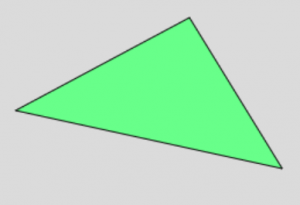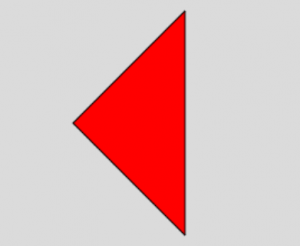# p5.js | triangle() Function

The triangle() function is an inbuilt function in p5.js which is used to draw a triangle in a plane. This function accepts three vertices of triangle.

Syntax:

`triangle(x1, y1, x2, y2, x3, y3)`

Parameters: This function accepts six parameters as mentioned above and described below:

• x1: This parameter accept the x-coordinate of first point.
• y2: This parameter accept the y-coordinate of first point.
• x2: This parameter accept the x-coordinate of second point.
• y2: This parameter accept the y-coordinate of second point.
• x3: This parameter accept the x-coordinate of third point.
• y3: This parameter accept the y-coordinate of third point.

Below programs illustrates the triangle() function in p5.js:

• Program 1:

 `function` `setup() { ` `    ``createCanvas(400, 400); ` `} ` ` `  `function` `draw() { ` `    ``background(220); ` `    ``fill(``'lightgreen'``); ` ` `  `    ``// A triangle at (100, 250), (250, 170) and (330, 300)   ` `    ``triangle(100, 250, 250, 170, 330, 300);  ` `} `

Output:• Program 2:

 `function` `setup() { ` `    ``createCanvas(400, 400); ` `} ` ` `  `function` `draw() { ` `    ``background(220); ` `    ``fill(``'red'``); ` `     `  `    ``// A triangle at (200, 100), (100, 200) and (200, 300)   ` `    ``triangle(200, 100, 100, 200, 200, 300);  ` `} `

Output:Reference: https://p5js.org/reference/#/p5/triangleMy Personal Notes arrow_drop_upCheck out this Author's contributed articles.

If you like GeeksforGeeks and would like to contribute, you can also write an article using contribute.geeksforgeeks.org or mail your article to contribute@geeksforgeeks.org. See your article appearing on the GeeksforGeeks main page and help other Geeks.

Please Improve this article if you find anything incorrect by clicking on the "Improve Article" button below.